# Samacheer Kalvi 8th Science Solutions Term 1 Chapter 3 Light

## Tamilnadu Samacheer Kalvi 8th Science Solutions Term 1 Chapter 3 Light

### Samacheer Kalvi 8th Science Light Text Book Exercises

8th Science Light Question And Answer Question 1.

Mirrors having a curved reflecting surface are called as –
(a) Plane mirrors
(b) Spherical mirrors
(c) Simple mirrors
(d) None of the above
(b) Spherical mirrors

8th Std Science Light Lesson Question 2.
The spherical mirror with a reflecting surface curved inward is called –
(a) Convex mirror
(b) Concave mirror
(c) Curved mirror
(d) None of the above
(b) Concave mirror

8th Standard Science Light Lesson Question 3.
The centre of a sphere of which the reflecting surface of a spherical mirror is a part is called –
(a) Pole
(b) Centre of curvature
(d) Aperture
(b) Centre of curvature

Samacheer Kalvi Guru 8th Science Question 4.
The spherical mirror used as a rear view mirror in the vehicle is –
(a) Concave mirror
(b) Convex mirror
(c) Plane mirror
(d) None of the above
(b) Convex mirror

Samacheer Kalvi 8th Science Book Solutions Question 5.
The imaginary line passing through the centre of curvature and pole of a spherical mirror is called –
(a) Centre of curvature
(b) Pole
(c) principal axis
(c) principal axis

Samacheerkalvi.Guru 8th Science Question 6.
The distance from the pole to the focus is called –
(a) Pole length
(b) Focal length
(c) principal axis
(d) None of the above
(b) Focal length

Samacheer Kalvi Guru Science 8th Standard Question 7.
Focal length is equal to half of the –
(a) Centre of curvature
(b) Axis
(d) None of the above

Samacheer Kalvi Guru 8 Science Question 8.
If the focal length of a spherical mirror is 10 cm, what is the value of its radius of curvature?
(a) 10 cm
(b) 5 cm
(c) 20 cm
(d) 15 cm
(c) 20 cm

Samacheer Guru 8th Science Question 9.
If the image and object distance is same, then the object is placed at –
(a) Infinity
(b) At F
(c) Between f and P
(d) At C
(d) At C

Samacheer Kalvi Guru 8th Standard Science Question 10.
The refractive index of water is –
(a) 1.0
(b) 1.33
(c) 1.44
(d) 1.52
(b) 1.33

II. Fill in the blanks

1. The spherical mirror used in a beauty parlour as make – up mirror is ………………
2. Geometric centre of the spherical mirror is ………………
3. Nature of the images formed by a convex mirror is ………………
4. The mirror used by the ophthalmologist to examine the eye is ………………
5. It the angle of incidence is 45°, then the angle of reflection is ………………..
6. Two mirrors are parallel to each other, then the number of images formed is ………………

1. Concave mirror
2. pole
3. Smaller, virtual and erect
4. Concave mirror
5. 45°
6. Infinite

III. Match the following

Question A.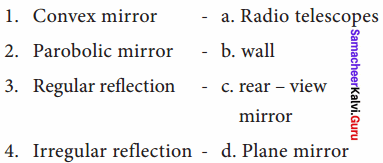1. c
2. a
3. d
4. b

Question B.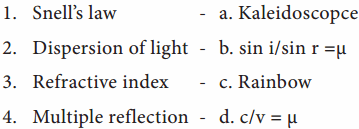1. b
2. c
3. d
4. a

Samacheer Kalvi Guru 8th Science Book Solutions Question 1.
What is called a spherical mirror?
Spherical mirrors are one form of curved mirrors. If the curved mirror is a part of a sphere, then it is called a ‘spherical mirror’.

8th Standard Light Lesson Question 2.
Define focal length.
The distance between the pole and the principal focus is called focal length (f) of a spherical mirror.

8th Science Solutions Samacheer Kalvi Question 3.
The radius of curvature of a spherical mirror is 25 cm. Find its focal length.
Given : Radius of curvature = 25 cm
To find: f = ?
Solution:
f = $$\frac { R }{ 2}$$ = $$\frac { 25 }{ 2 }$$
f = 12.5 cm

Kalvi Guru 8th Science Question 4.
Give two applications of a concave and convex mirror.
Concave mirrors:

1. Concave mirrors are used while applying make – up or shaving, as they provide a magnified image.
2. They are used in torches, search lights and head lights as they direct the light to a long distance.

Convex mirrors:

1. Convex mirrors are used in vehicles as rear view mirrors because they give an upright image and provide a wider field of view as they are curved outwards.
2. They are found in the hallways of various buildings including hospitals, hotels, schools and stores. They are usually mounted on a wall or ceiling where hallways make sharp turns.

Samacheer Kalvi 8th Science Book Solutions Term 1 Question 5.
State the laws of reflection.

1. The incident ray, the reflected ray and the normal at the point of incidence, all lie in the same plane.
2. The angle of incidence and the angle of reflection are always equal.

Samacheerkalvi.Guru Science 8th Question 6.
If two plane mirrors are inclined to each other at an angle of 45°, find the number of images formed.
Given :
Angle of inclination = 45°
To find : Number of images formed = $$\frac { 360° }{ angle }$$ – 1
Solution:
= $$\frac { 360° }{ 45% }$$ – 1
= 8 – 1
= 7 images

Samacheer Class 8 Science Solutions Question 7.
Define the refractive index of a medium.
The amount of refraction of light in a medium is denoted by a term known as refractive index of the medium, which is the ratio of the speed of light in the air to the speed of light in that particular medium.

Light Class 8 Questions And Answers Pdf Question 8.
State the Snell’s law of refraction.
Refraction of light rays, as they travel from one medium to another medium, obeys two laws, which are known as Snell’s laws of refraction. They are:

1. The incident ray, the refracted ray and the normal at the point of intersection, all lie in the same plane.

2. The ratio of the sine of the angle of incidence (i) to the sine of the angle of refraction (r) is equal to the refractive index of the medium, which is a constant.
$$\frac { sin i }{ sin r}$$ = µ

Light Class 8 Questions And Answers Question 1.
Explain the images formed by a concave mirror?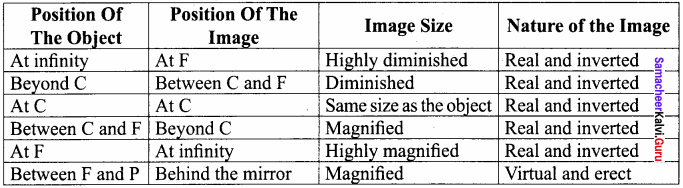Samacheer Kalvi 8th Tamil Book Solutions Question 2.
What is reflection? Write short notes on regular and irregular reflection?
A ray of light, falling on a body having a shiny polished and smooth surface alone is bounced back. This bouncing back of the light rays as they fall on the smooth, shiny and polished surface is called reflection.
Regular reflection:

1. When a beam of light (collection of parallel rays) falls on a smooth surface, it gets reflected.
2. After reflection, the reflected rays will be parallel to each other. Here, the angle of incidence and the angle of reflection of each ray will be equal.
3. Hence, the law of reflection is obeyed in this case and thus a clear image is formed. This reflection is called ‘regular reflection’ or ‘specular reflection’.

Irregular reflection:

1. In the case of a body having a rough or irregular surface, each region of the surface is inclined at different angles.
2. When light falls on such a surface, the light rays are reflected at different angles.
3. In this case, the angle of incidence and the angle of reflection of each ray are not equal.
4. Hence, the law of reflection is not obeyed in this case and thus the image is not clear. Such a reflection is called ‘irregular reflection’ or ‘diffused reflection’.

Samacheer Kalvi 8th Books Solutions Science Question 3.
Explain the working of a periscope.
Periscope:

1. It is an instrument used for viewing bodies or ships, which are over and around another body or a submarine.
2. It is based on the principle of the law of reflection of light.
3. It consists of a long outer case and inside this case mirrors or prisms are kept at each end, inclined at an angle of 45°.
4. Light coming from the distant body, falls on the mirror at the top end of the periscope and gets reflected vertically downward.
5. This light is reflected again by the second mirror kept at the bottom, so as to travel horizontally and reach the eye of the observer.
6. In some complex periscopes, optic fibre is used instead of mirrors for obtaining a higher resolution.
7. The distance between the mirrors also varies depending on the purpose of using

Samacheerkalvi.Guru 8th Question 4.
What is dispersion? Explain in detail.

1. Splitting of white light into its seven constituent colours (wavelength), on passing through a transparent medium is known as dispersion of light.
2. Dispersion occurs because, light of different colours present in white light have different wavelength and they travel at different speeds in a medium.
3. Refraction of a light ray in a medium depends on its speed.

Light Question Answer Class 8 Question 5.
Speed of light in air is 3 x 108 m s-1 and the refractive index of a medium is 1.5. Find the speed of light in the medium.
Given :
Speed of light in air c = 3 x 108 ms-1
Refractive index of a medium µ = 1.5
To find : Speed of light in medium v = ?
Formula :
µ = $$\frac { c }{ v}$$
Solution:
1.5 = $$\frac{3×10^{8}}{v}$$
v =$$\frac{3×10^{8}}{1.5}$$
v = 2 x 108 ms-1
Speed of light in medium v = 2 x 108 ms-1

### Samacheer Kalvi 8th Science Solutions Light Additional Questions

Class 8 Science Chapter 3 Solution Question 1.
Which object use the reflection of light?
(a) Kaleidoscope
(b) Plane mirror
(c) Convex mirror
(d) All of these
(d) All of these

Class 8 Science Light Question 2.
Which surface will not reflect most of the light falling on them?
(a) Rough surface
(b) Smooth surface
(c) Shining surface
(d) Opaque surface
(a) Rough surface

Question 3.
Which of the following demonstrates the law of reflection?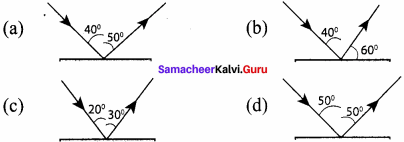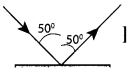Question 4.
The ENT doctor uses a …………….
(a) Plane mirror
(b) Concave mirror
(c) Convex mirror
(d) Convex lens
(b) Concave mirror

Question 5.
In dispersion, the colour of light that will bend more is ……………..
(a) Red
(b) yellow
(c) Green
(d) Violet
(d) violet

Question 6.
Reflection by a looking mirror is called …………….
(a) Regular reflection
(b) Irregular reflection
(c) Regular and irregular reflection
(d) None of these
(a) Regular reflection

Question 7.
The velocity of light in vacuum is 3 x 108 ms-1 and in glass is 2 x 108 ms-1. The refractive index of glass is .
(a) 2
(b) 1.5
(c) 1.8
(d) 1.33
(b) 1.5

Question 8.
Incident angle of a ray of light is 30°. The angle between the incidend ray and the reflected ray is …………….
(a) 50°
(b) 90°
(c) 60°
(d) 15°
(c) 60°

Question 9.
In the head lights of motor vehicles, ……………… mirrors are used as reflectors.
(a) Plane mirrors
(b) Concave lenses
(c) Convex mirrors
(d) Concave mirrors
(d) Concave mirrors

Question 10.
The phenomenon of light passing through the object is called ……………..
(a) Reflection
(b) Refraction
(c) Dispersion
(d) Total internal reflection
(b) Refraction

II. Fill in the Blanks

1. The bouncing back of light from a surface is called …………….
2. ……………. mirrors make things look larger when objects are placed close to it
3. Convex mirror always forms ……………. and ……………. image.
4. The incident ray, ……………. ray and the ……………. at the point of incidence, all lie on the same plane.
5. A ray of light incident along normal to the mirror ……………. its path.
6. When light passes from one medium to another the ray gets bent. This property of light is called ……………..
7. Spherical mirrors are one form of …………… mirrors.
8. ……………… mirrors magnify the object placed close to them.
9. The image formed by convex mirrors is ……………… than the object
10. …………….. mirrors form the perfect image of an object.
11. The …………….. of a mirror determines the type of image it forms.
12. The ……………. is an optical device with a polished surface that reflects the light falling on it.

1. Reflection
2. Concave
3. Virtual and erect
4. Reflected, normal
5. Retraces
6. Refraction
7. Curved
8. Concave
9. Smaller
10. Plane
11. Shape
12. Mirror

III. True or False – if false give the correct statement

Question 1.
We can see things around us only when the light reflected by them reaches our eyes.
True.

Question 2.
Light is a form of energy and it travels in a straight line.
True.

Question 3.
The periscope is an optical device with a polished surface that reflects the light falling on it.
False.
Correct statement:
The mirror is an optical device with a polished surface that reflects the light falling on it.

Question 4.
Curved mirrors have surfaces that are spherical, cylindrical, parabolic and ellipsoid.
True.

Question 5.
Curved mirrors form the perfect image of an object.
False. Correct statement: Plane mirrors form the perfect image of an object.

Question 6.
Curved mirrors produce images that are either enlarged or diminished.
True.

Question 7.
A thin layer of molten aluminium or silver is used for coating glass plates that will then become mirrors.
True.

Question 8.
The most common example of a convex mirror is the make – up mirror.
False.
Correct statement:
The most common example of a concave mirror is the make – up mirror.

IV. Match the following

Question 1.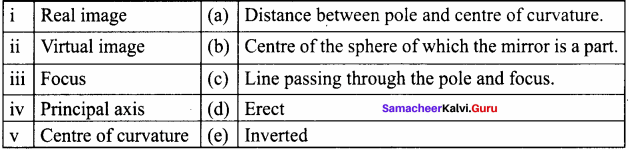i. e
ii. d
iii. a
iv. c
v. b

Question 2.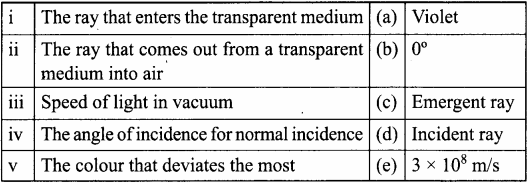i. e
ii. d
iii. a
iv. c
v. b

Question 3.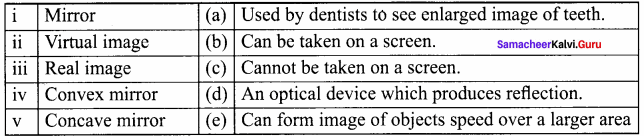i. d
ii. c
iii. b
iv. e
v. a

V. Assertion and Reason

Mark the correct choice as:
(a) If both assertion and reason are true and the reason is the correct explanation of the assertion.
(b) If both assertion and reason are true, but the reason is not the correct explanation of the assertion.
(c) If the assertion is true, but the reason is false.
(d) If the assertion is false, but the reason is true.

Question 1.
Assertion : A ray incident along normal to the mirror retraces its path
Reason : In reflection, angle of incidence is always equal to angle of reflection.
(a) Both assertion and reason are true and the reason is the correct explanation of the assertion

Question 2.
Assertion : Convex mirrors are used as rear view mirror in vehicles for observing traffic at our back.
Reason : A convex mirror has a much larger field of view.
(a) Both assertion and reason are true and the reason is the correct explanation of the assertion

Question 3.
Assertion : The mirrors used in search lights are parabolic and not concave spherical.
Reason : In concave spherical mirror the image formed is always virtual.
(c) The assertion is true, but the reason is false
Correct explanation:
In search lights, we need an intense parallel beam of light. If a source is placed at the focus of a concave spherical mirror only paraxial rays are rendered parallel. Due to large aperture of mirror, marginal rays give a divergent beam. But in case of parabolic mirror, when source is at the focus, beam of light produced over the entire cross – section of the mirror is a parallel beam.

Question 4.
Assertion : We can see the rainbow in the sky when the rain starts falling after a spell of bright sunlight.
Reason : The rainbow is formed due to the dispersion of light.
(d) The assertion is false, but the reason is true
Correct explanation:
The rainbow is formed when the light passes through the water droplets in air after it rains and gets dispersed into seven colours.

Question 1.
How does the light travel?
The light travels along straight lines.

Question 2.
What is reflection of light?
The bouncing back of light when it falls on smooth surface is called reflection.

Question 3.
What is mirror?
The mirror is an optical device with a polished surface that reflects the light falling on it.

Question 4.
What type of image is formed by a concave mirror?
Real and inverted image. If the object is placed very close to the mirror then the image is virtual and erect.

Question 5.
What is rainbow?
The rainbow is seen as a large area in the sky with many colours.

Question 6.
Name the triangular piece of glass that splits white light into different colours.
Prism.

Question 7.
What is the composition of sunlight?
Sunlight is a mixture of seven colours.

Question 8.
Light bends as it passes from one medium to another. What is this phenomenon called?
Refraction of light.

Question 9.
Name the two types of spherical mirrors.

1. Concave mirror
2. Convex mirror.

Question 10.
The angle between incident ray and reflected ray is 60°. What is the value of angle of incidence?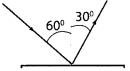since angle of incidence = angle of reflection.
So, angle of incidence = 30°.

Question 1.
Light travels fastest in vacuum. Why?
Light travels fastest in vacuum than any other medium because there is no obstruction to the passage of light in vacuum.

Question 2.
Distinguish between real and virtual images.
Real image:
Type of image which can be obtained on a screen is called a real image

Virtual image:
An image which cannot be obtained on a screen is called a virtual image.

Question 3.
State any two uses of concave mirrors.

1. It is used as a reflector in torches, light houses, head lights of vehicles, etc., as it diverges the rays of light.
2. A dentist uses a concave mirror to obtain a magnified image of the teeth of the patient.

Question 4.
A convex rear view mirror is preferred over a plane mirror in a car. Why?
Since convex mirror forms a smaller and virtual image, it can be used to see a much larger area than the area visible by a plane mirror.

Question 5.
What type of image is formed by a concave mirror?
The image formed by a concave mirror is real and inverted. If the object is placed very rear to the mirror then the image formed is virtual and erect.

Question 6.
Why do we need a shiny surface for reflection?
The extent of reflection depends upon the shine and smoothness of the surface. More is the shine and smoothness of the surface more will be the reflection.

Question 7.
The radius of curvature of a spherical mirror is 18 cm. What is the focal length of this mirror?
f = $$\frac { R}{ 2 }$$
R = 18 cm
f = $$\frac { 18}{ 2 }$$ = 9 cm.

Question 8.
What happens to light when it gets dispersed? Give an example.
Light is splitted into its constituent colours, when it gets dispersed.
Example:
Rainbow formation is due to the dispersion of white light after passing through water droplets.

Question 9.
If two mirrors are placed at an inclination of 30° then how many images can be seen?
Formula : Number of images N = $$\frac { 360°}{ 30° }$$ – 1
Given: θ = 30°
Solution:
N = $$\frac { 360°}{ 30° }$$ – 1
= 12 – 1 = 11 images.

Question 10.
What is the speed of light in diamond if its refractive index is 2.41?
Formula :
Refractive index µ =$$\frac {Speed of light in air (c) }{ Speed of light in the medium (v) }$$
Given : µ = 2.41
Solution:
c = 3 x 108ms-1
µ = $$\frac { c }{ v }$$
v =$$\frac{3×10^{8}}{2.41}$$
Speed of light in diamond v = 1.24 x 108 ms-1

Question 11.
A light ray moves from glass (Vglass = 2.0 x 108ms-1) to diamond (Vdiamond = 1.25 x 108 ms-1). What is the refractive index of diamond with respect to glass?
Refractive index of diamond with respect to glass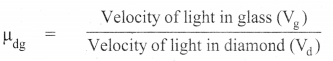Solution: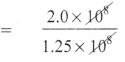= $$\frac { 200 }{ 125 }$$
= 1.60 (No unit).

Question 12.
Find the refractive index of water with respect to glass if the refractive index of water is $$\frac { 4 }{ 3 }$$ and the reiractive index of glass is $$\frac { 3 }{ 2 }$$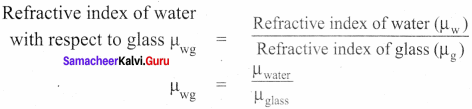Solution:
= $$\frac { 4 }{ 3 }$$ x $$\frac { 2}{ 3 }$$
= $$\frac { 8 }{ 9}$$
Thus, refractive index
of water with respect to glass = $$\frac { 8 }{ 9}$$ (No unit).

Question 1.
Differentiate between regular and irregular reflection.
Regular reflection:

1. When a beam of light (collection of parallel rays) falls on a smooth surface, it gets reflected. After reflection, the reflected rays will be parallel to each other.
2. Here, the angle of incidence and the angle of reflection of each ray will be equal.
3. Hence, the law of reflection is obeyed in this case and thus a clear image is formed.
4. This reflection is called ‘regular reflection’ or ‘specular reflection’.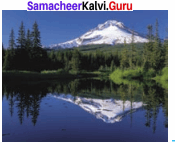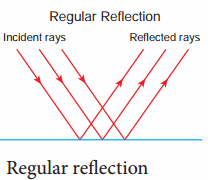Example : Reflection of light by a plane mirror and reflection of light from the surface of still water.

Irregular reflection:

1. In the case of a body having a rough or irregular surface, each region of the surface is inclined at different angles.
2. When light falls on such a surface, the light rays are reflected at different angles. In this case, the angle of incidence and the angle of reflection of each ray are not equal.
3. Hence, the law of reflection is not obeyed in this case and thus the image is not clear. Such a reflection is called ‘irregular reflection’ or ‘diffused reflection’.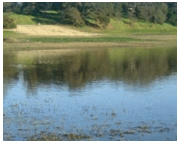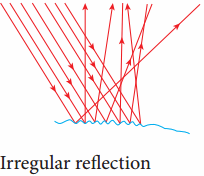Example : Reflection of light from a wall.

Question 2.
Explain the construction and working of kaleidoscope.
Construction:

1. Take three equal sized plane mirror strips and arrange them in such a way that they form an equilateral triangle.
2. Cover the sides of the mirrors with a chart paper. With the help of a chart paper cover the bottom of the mirrors also.
3. Put some coloured things such as pieces of bangles and beads inside it.
4. Now, cover the top portion with the chart paper and make a hole in it to see.
5. You can wrap the entire piece with coloured papers to make it attractive.
6. Now, rotate it and see through its opening. You can see the beautiful patterns.

Question 3.
Explain the construction, working of periscope with a neat labelled diagram.

1. It is an instrument used for viewing bodies or ships, which are over and around another body or a submarine.
2. It is based on the principle of the law of reflection of light. It consists of a long outer case and inside this case mirrors or prisms are kept at each end, inclined L’9ht at an angle of 45°.
3. Light coming from the distant body, falls on the mirror at the top end of the periscope and gets reflected 450 vertically downward.
4. This light is reflected again by the second mirror kept at the bottom, so as to travel horizontally and reach the eye of the observer.
5. In some complex periscopes, optic fibre is used instead of mirrors for obtaining a higher resolution.
6. The distance between the mirrors also varies depending on the purpose of using the periscope.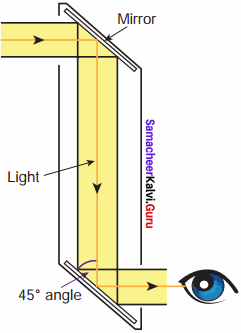Question 4.
Explain the uses of periscope.

1. It is used in warfare and navigation of the submarine.
2. In military it is used for pointing and firing guns from a ‘bunker’.
3. Photographs of important places can be taken through periscopes without trespassing restricted military regions.
4. Fibre optic periscopes are used by doctors as endoscopes to view internal organs of the body.

Question 5.
Explain the images formed by a convex mirror for different position of the object.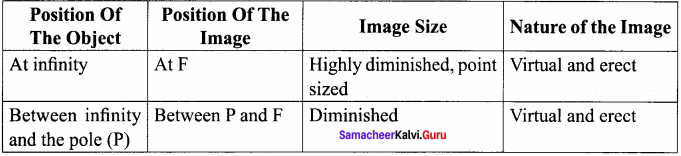Question 6.
1. A pencil placed in water in a glass appear to be bent at the surface of separation.

2. The base of a swimming pool appears to be raised due to refraction of light.

3. We should look straight down into the river while catching fish. If observed obliquely, the fish appears to be closer to the surface of the water than it actually is.

4. Stars look like tiny dots when viewed from earth, because they are far away from us. The light from the stars has to travel a long distance through the atmosphere before it reaches us. The atmosphere has various layers of different refractive indices. Light is refracted through each of them. These layers are turbulent. Every time one of the layers shifts, the thickness and refractive indices of air change and the light is refracted differently, creating a twinkling effect.

IX. Complete the given table

Question 1.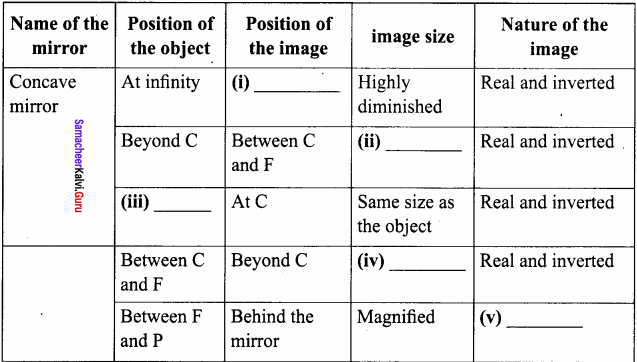1. At F
2. Diminished
3. At C
4. Magnified
5. Virtual and erect

X. Draw the following

Question 1.
Concave mirror.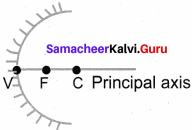Question 2.
Convex mirror.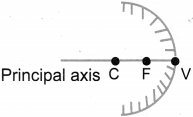Question 3.
Draw the ray diagram and write the characteristics of the image formed when an object is placed

1. At infinity in front of a concave mirror.
2. At infinity in front of a convex mirror.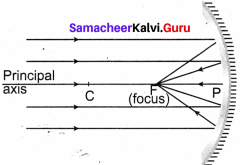Position of the image : At F
Image size : Highly diminished
Nature of the image : Real and inverted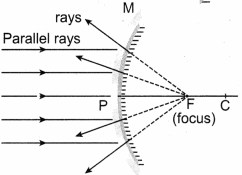Position of the image : At F
Image size : Highly diminished , point sized
Nature of the image : Virtual and erect

Question 4.
Draw a neat labelled diagram of a periscope.Question 5.
Draw a ray diagram to show a light ray travels from denser medium (glass) to rarer medium (air).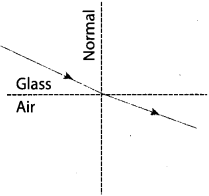When a light ray travels from denser medium to rarer medium, it speeds up and refracts away from the normal.

Question 6.
Draw a ray diagram to show a light ray travels from rarer medium (air) to denser medium (water).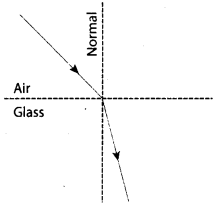When a light ray travels from rarer to denser medium, it bends towards the normal.

XI. Cross word puzzle

Across:
3. The geometrical centre of a spherical mirror.
6. Centre of the sphere from which the mirror is made.
7. An optical device with a polished surface that reflects the light falling on it.
8. Image which can be formed on a screen.
9. Image which cannot be formed on a screen.
10. The bending of a light ray when it passes from one medium to another medium of different density.

Down:
1. Mirror which converges a parallel beam of light passing through it.
2. Imaginary line passing through the centre of curvature of the mirror.
4. Mirror which diverges a parallel beam of light passing through it.
5. The formation of rainbow is an example of ______.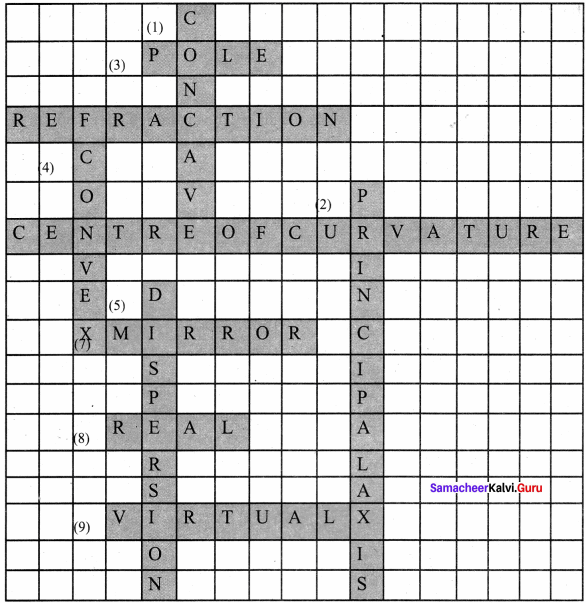Across:
3. Pole
6. Centre Of Curvature
7. Mirror
8. Real
9. Virtual
10. Refraction

Down:
1. Concave
2. Principal Axis
4. Convex
5. Dispersion

XI. Creative questions : HOTS

Question 1.
Imagine that parallel rays are incident on an irregular surface. Are the rays reflected from the surface parallel to each other?
No, the reflected rays from irregular surface are in different direction.

Question 2.
A safety vest helps to keep the workers who are working by the roadside safe. This especially so during the nights. Why?
The reflectors on the safety vest reflect light into the motorists eyes.
This help to alert the motorists of the wearer’s presence on the road.

Question 3.
What is the difference between virtual images of an object formed by a concave mirror and a convex mirror?
The virtual image of an object formed by a concave mirror is always magnified one but the image formed by a convex mirror is always diminished one.

Question 4.
What is a virtual image? Give one situation where a virtual image is formed.
The image formed by the plane mirror appears behind it. We cannot however touch it. Also, the image of the object cannot be obtained on a screen, whether it is held in front of the mirror or behind it. Such type of images are not real. They are virtual images.

Question 5.
If all objects around us were to reflect light in a regular way, what problem might we face?